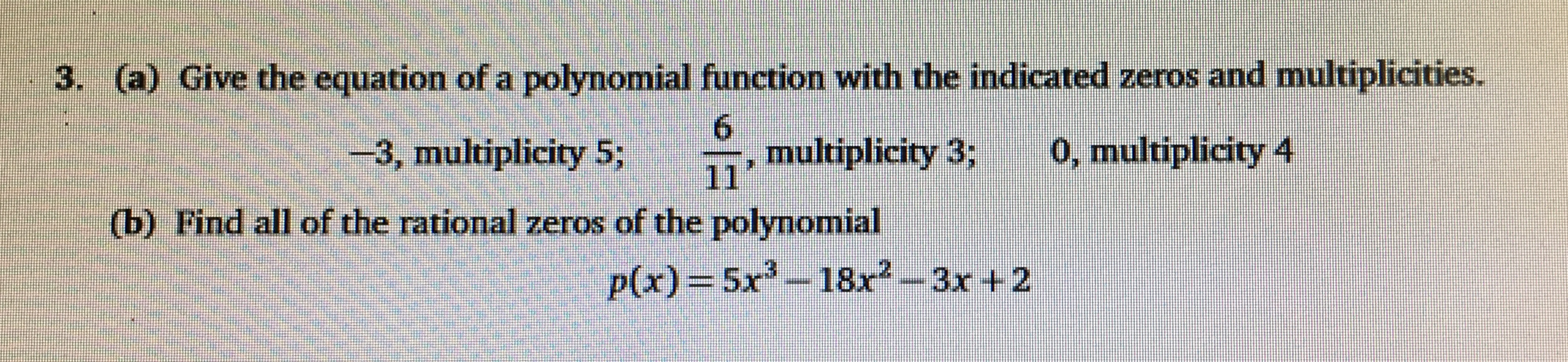# 3. (a) Give the equation of a polynomial function with the indicated zeros and multiplicities. 6 multiplicity 3; 0, multiplicity 4 3, multiplicity 5; 111 (b) Find all of the rational zeros of the polynomial p(x)=5x-18x2--3x +2

Questionhelp_outlineImage Transcriptionclose3. (a) Give the equation of a polynomial function with the indicated zeros and multiplicities. 6 multiplicity 3; 0, multiplicity 4 3, multiplicity 5; 111 (b) Find all of the rational zeros of the polynomial p(x)=5x-18x2--3x +2 fullscreen

### Want to see this answer and more?

Experts are waiting 24/7 to provide step-by-step solutions in as fast as 30 minutes!*

*Response times vary by subject and question complexity. Median response time is 34 minutes and may be longer for new subjects.
Tagged in
MathCalculus

### Other